Home > CC4 > Chapter 5 > Lesson 5.2.3 > Problem5-80

5-80.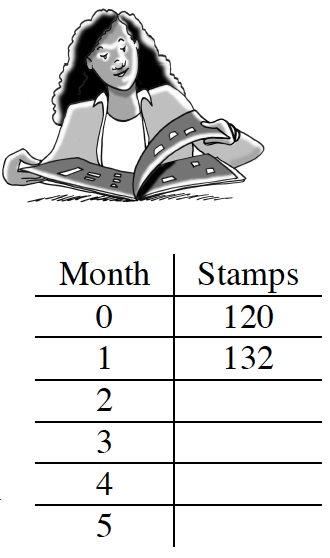Lona received a stamp collection from her grandmother. The collection is in a leather book and currently has 120 stamps. Lona joined a stamp club, which sends her 12 new stamps each month. The stamp book holds a maximum of 500 stamps. Homework Help ✎

1. Complete the table at right.

2. How many stamps will Lona have in one year from now?

3. Write an equation using function notation to represent the total number of stamps that Lona has in her collection after n months. Let the total be represented by t(n).

4. Solve your equation from part (c) for n when t(n) = 500. Will Lona be able to fill her book exactly with no stamps remaining? How do you know? When will the book be filled?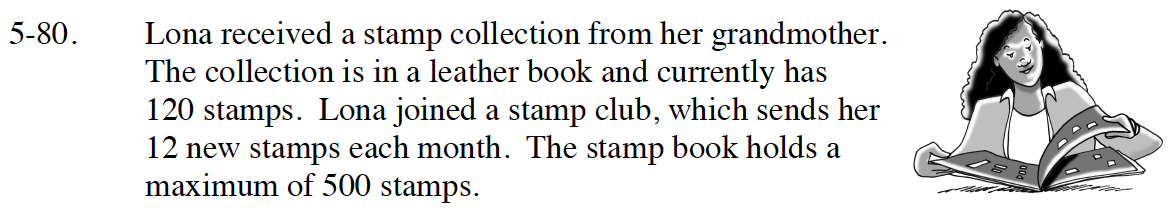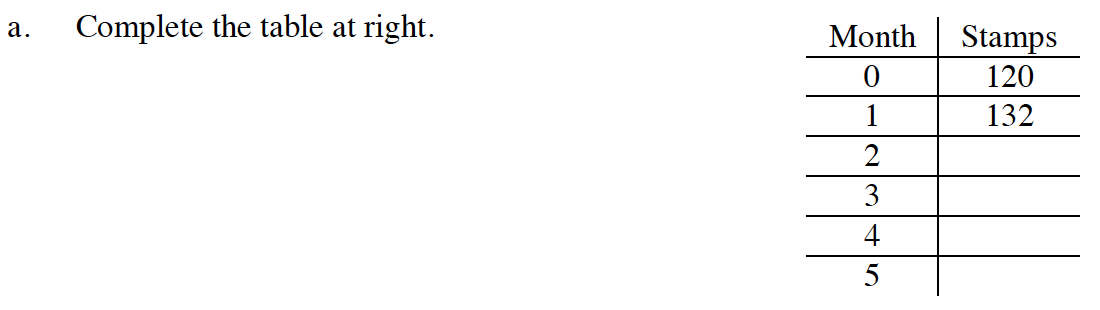Continue adding 12 to find months 2-5.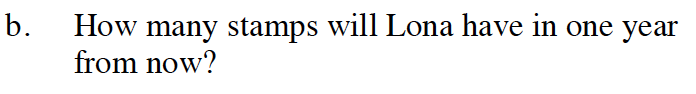Continue the table to month 12.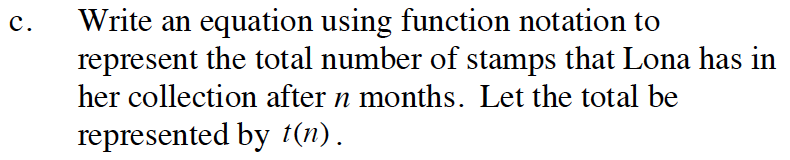Remember that the initial amount was 120 stamps and the rate is 12 stamps per month.

t(n) = 120 + 12n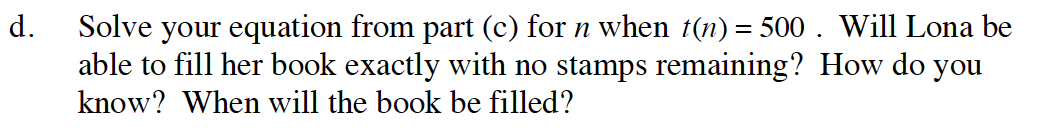Substitute 500 for t(n).

500 = 120 +12n